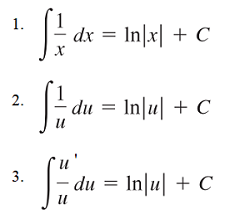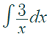# Log Rule for Integration

## Log Rule for Integration: Definition

The log rule for integration can be written in three different ways:Log rule for integration: With x as a variable (1) or change of variables u (2). An alternate form (3) is allowed because du = u′ dx .

Which rule you use depends on what is in your denominator.

1. Rule 1: x in the denominator. Example 1.
2. Rule 2: An equation with x in the denominator, like “x2 – 2″ or “5x3 + 10″: Example 2.

## Examples

Example question #1 (x as the variable): Find the integral ofSolution:
Step 1: Write out the right hand side of the rule:
We’re using rule (1) for this example, so:
= ln |x| + C

Note: The || symbol is for the absolute value).

Step 2: Take the constant in the numerator (because of the constant rule for integration) of the expression and place it in front of the equation you wrote in Step 1. We have a constant of 3, so:
= 3 ln |x|

= 3 ln |x| + C

That’s it!

Note: We can move the constant in front because of the constant rule of integration, which states ∫a · f(x) dx = a ∫f(x) dx.

Example question #2 (change of variables):
Change of variables means that you can switch out an expression for “u” to make integrating easier.

Example question: Find the integral of:Step 1: Substitute the expression in the denominator for “u” and substitute “du” for “dx”*.*”dx” means “a small change in x”. Because you’ve replaced the x in the denominator for u, you’re now looking for a small change in u.

Step 2: Take the constant out (because we need to get part of the expression looking exactly like rule #2):Step 3: Use rule 2 to rewrite the expression:Step 4: Substitute the original expression back in for “u”:Step 5: Add a + C:That’s it!

## Log Rule for Integration vs. General Rule

Despite the similar sounding names, the log rule for integration is completely different from the general rule for the integral of natural log :

∫ ln(x)dx = x · ln(x) – x + C.

This can get a little confusing because of the similar sounding name. However, the important thing is that with the “general rule”, you’re integrating a logarithm. In other words, the question contains the natural logarithm. With the log rule for integration, you’re integrating a function f(x) = 1/x; the solution contains a natural logarithm.

## References

 Larson, R. & Edwards, B. (2016). Calculus, 10th Edition. Cengage Learning.
 Aspnes, J. (2021). HowToIntegrate. Retrieved April 18, 2021 from: https://www.cs.yale.edu/homes/aspnes/pinewiki/HowToIntegrate.html

CITE THIS AS:
Stephanie Glen. "Log Rule for Integration" From StatisticsHowTo.com: Elementary Statistics for the rest of us! https://www.statisticshowto.com/log-rule-for-integration/